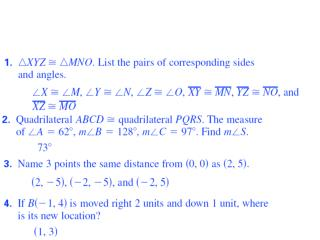DownloadDownload Presentation7.1 Rigid Motion in a Plane

# 7.1 Rigid Motion in a Plane

Download Presentation## 7.1 Rigid Motion in a Plane

- - - - - - - - - - - - - - - - - - - - - - - - - - - E N D - - - - - - - - - - - - - - - - - - - - - - - - - - -
##### Presentation Transcript

1. 7.1 Rigid Motion in a Plane Geometry, NCSCOS: 3.01 Describe the transformation (translation, reflection, rotation, dilation) of polygons in the coordinate plane in simple algebraic terms.

2. Objective: • Students will understand geometric concepts and applications. • Describe the effect of rigid motions on figures in the coordinate plane and space that include rotations, translations, and reflections • Identify the three basic rigid transformations. • Students will have different activities related with the topic hands on or worksheet after ppt. • (Keep in consideration 30min for completing the test for CH6)

3. Essential Question: • How do we identify the three basic rigid transformations in a coordinate plane?

4. Identifying Transformations • Figures in a plane can be • Reflected • Rotated • Translated to produce new figures. The new figure is called the IMAGE. The original figures is called the PREIMAGE. The operation that MAPS, or moves the preimage onto the image is called a transformation.

5. Which are basic transformations? • Three basic transformations: • Reflections • Rotations • Translations • And combinations of the three. • For each of the three transformations on the next slide, the blue figure is the preimage and the red figure is the image. We will use this color convention throughout the rest of the book.

6. Examples: Rotation about a point Reflection in a line Translation

7. Some facts • Some transformations involve labels. When you name an image, take the corresponding point of the preimage and add a prime symbol. For instance, if the preimage is A, then the image is A’, read as “A prime.”

8. http://members.cox.net/fathauerart/TessellationArt.html http://www.tessellations.org/eschergallery1thumbs.htm

9. Example 1: Naming transformations • Use the graph of the transformation at the right. • Name and describe the transformation. • Name the coordinates of the vertices of the image. • Is ∆ABC congruent to its image?

10. Example 1: Naming transformations • Name and describe the transformation. The transformation is a reflection in the y-axis. You can imagine that the image was obtained by flipping ∆ABC over the y-axis/

11. Example 1: Naming transformations • Name the coordinates of the vertices of the image. The cordinates of the vertices of the image, ∆A’B’C’, are A’(4,1), B’(3,5), and C’(1,1).

12. Example 1: Naming transformations • Is ∆ABC congruent to its image? Yes ∆ABC is congruent to its image ∆A’B’C’. One way to show this would be to use the DISTANCE FORMULA to find the lengths of the sides of both triangles. Then use the SSS Congruence Postulate

13. ISOMETRY • An ISOMETRY is a transformation the preserves lengths. Isometries also preserve angle measures, parallel lines, and distances between points. Transformations that are isometries are called RIGID TRANSFORMATIONS.

14. Ex. 2: Identifying Isometries • Which of the following appear to be isometries? • This transformation appears to be an isometry. The blue parallelogram is reflected in a line to produce a congruent red parallelogram.

15. Ex. 2: Identifying Isometries • Which of the following appear to be isometries? • This transformation is not an ISOMETRY because the image is not congruent to the preimage

16. Ex. 2: Identifying Isometries • Which of the following appear to be isometries? • This transformation appears to be an isometry. The blue quadrilateral is rotated about a point to produce a congruent red quadrilateral.

17. Mappings • You can describe the transformation in the diagram by writing “∆ABC is mapped onto ∆DEF.” You can also use arrow notation as follows: • ∆ABC ∆DEF • The order in which the vertices are listed specifies the correspondence. Either of the descriptions implies that • A D, B E, and • C F.

18. Ex. 3: Preserving Length and Angle Measures • In the diagram ∆PQR is mapped onto ∆XYZ. The mapping is a rotation. Given that • ∆PQR ∆XYZ is an isometry, find the length of XY and the measure of Z. 35°

19. Ex. 3: Preserving Length and Angle Measures • SOLUTION: • The statement “∆PQR is mapped onto ∆XYZ” implies that P X, Q Y, and R Z. Because the transformation is an isometry, the two triangles are congruent. So, XY = PQ = 3 and mZ = mR = 35°. 35°

20. More Illustrations As you look at the image determine the type of translation. Art work Floor tiling ROTATION TRANSLATION http://library.thinkquest.org/16661/escher/tessellations.11.html http://www.camaraslate.com/images/flooring/E42601.jpg

21. More images Art work Stained Glass Window TRANSLATION REFLECTION http://www.easyboo.com/images/tb/IMG_3814a.JPG http://library.thinkquest.org/16661/escher/tessellations.11.html

22. More images Art work Quilting ROTATION REFLECTION http://library.thinkquest.org/16661/escher/tessellations.11.html www.lisettescountryfabriccreations.com

23. Transformations on your computer You can perform each of the transformations with the draw tool on your computer. One place to do this is in Word.

24. An example from Word Translation Rotation (copy and paste) (green circle and drag) Reflection (draw menu and flip horizontal)